# On Area percentage -3 (with bonus challenge!)

Not go on the title, in this discussion I will not deal with percentage but instead measure of area

In the below diagram $$O$$ is the center of the circle with tangents $$\overline{BD}$$ and $$\overline{AE}$$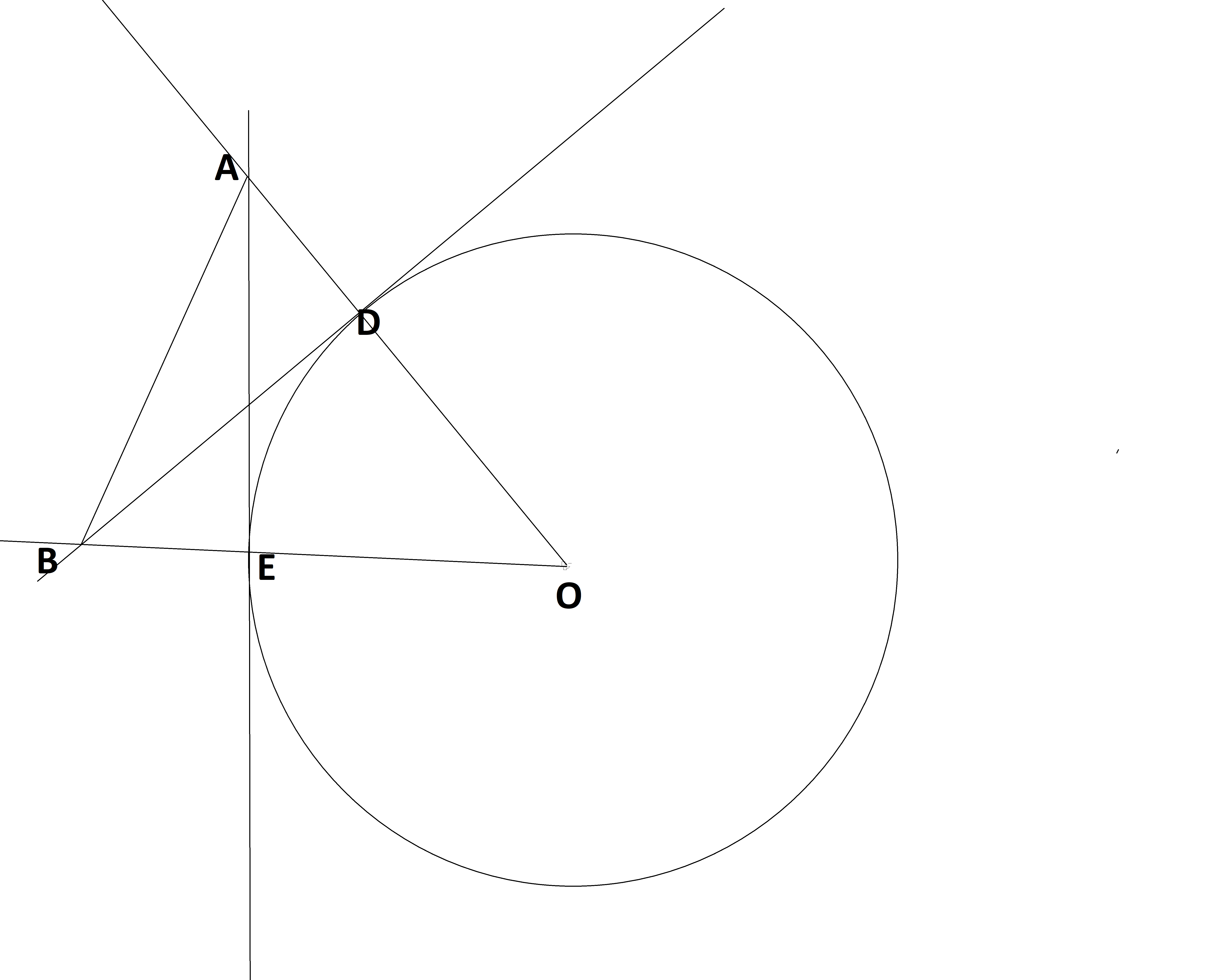The area of $\triangle AOB=\frac{1}{2}\overline{OD}^2\tan(\angle AOB)\sec(\angle AOB)$

Proof:

Let $\overline{OD}=x;\angle AOB=\theta$

In $\triangle AEO$ $\tan(\theta)=\dfrac{\overline{AE}}{x}$ $\overline{AE}=x\tan(\theta)........$ As $\angle AEO=90^\degree$, applying Pythagorus theorem $x^2+\overline{AE}^2=\overline{AO}^2$ $\overline{AO}^2=x^2+x^2\tan^2(\theta)=x^2(1+\tan^2(\theta))=x^2\sec^2(\theta)$ $\overline{AO}=x\sec(\theta)........$

Now in $\triangle AEO$ and $\triangle BDO$ $\angle AEO=90^\degree=\angle BDO$ $\angle AOE=\theta=\angle BOD$ $\overline{OE}=x=\overline{OD}$ From RHS criterion of congruence $\triangle AEO\cong\triangle BDO$ $\therefore \overline{AO}=\overline{BO}........$

Area of $\triangle ABO=\frac{1}{2}\overline{BO}\times\overline{AE}$

From $$ and this

Area of $\triangle ABO=\frac{1}{2}\overline{AO}\times\overline{AE}$

From $,$ and this

Area of $\triangle ABO=\frac{1}{2}x\sec(\theta)\times x\tan(\theta)=\frac{1}{2}x^2\tan(\theta)\sec(\theta)$

Bonus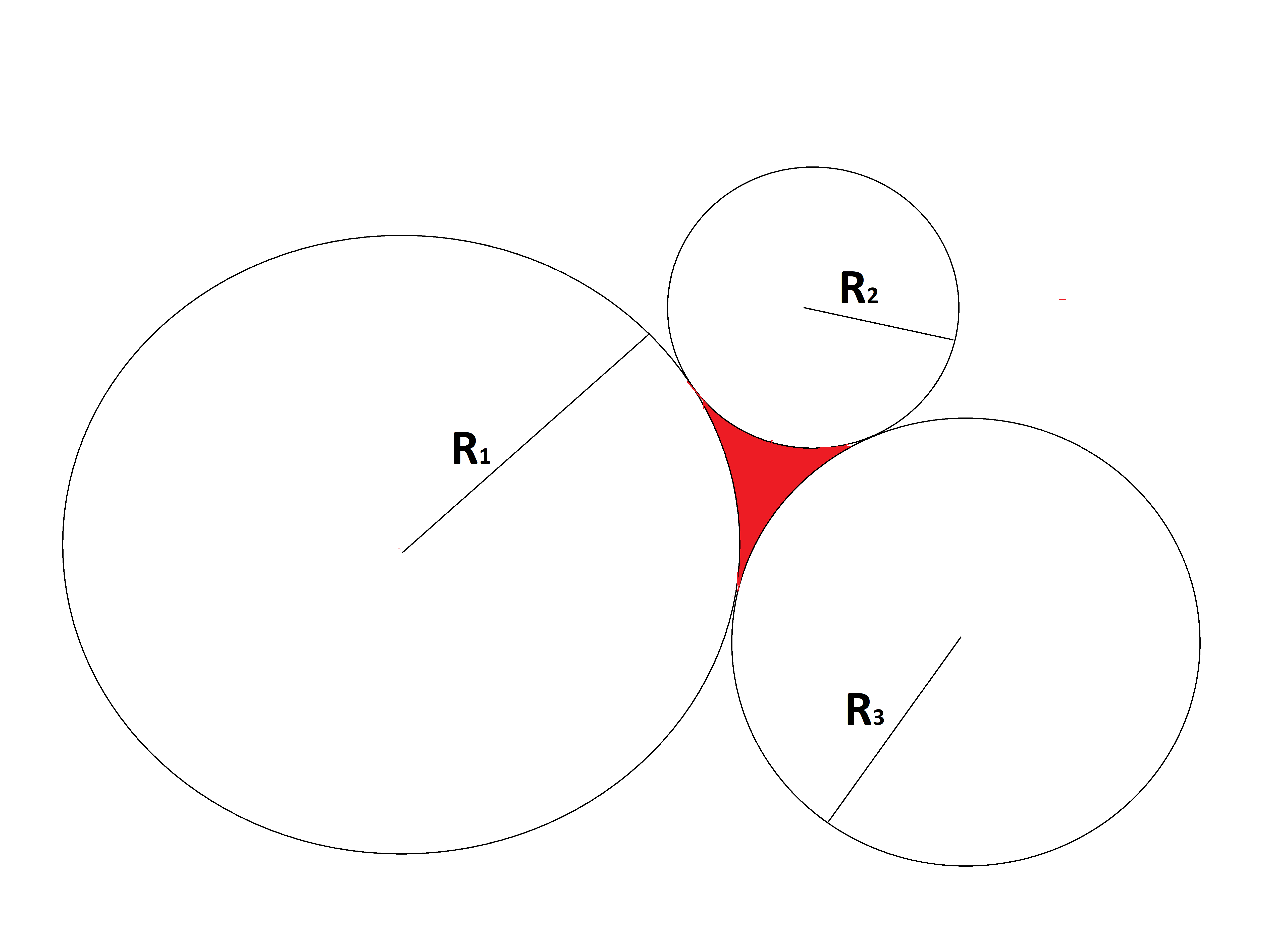In the above diagram if you know the value of $R_1,R_2$ and $R_3$, then find a formula to find the area of the $\red{red}$ region.

Note:

• I myself don't know the answer to the bonus problem.Note by Zakir Husain
1 year, 1 month ago

This discussion board is a place to discuss our Daily Challenges and the math and science related to those challenges. Explanations are more than just a solution — they should explain the steps and thinking strategies that you used to obtain the solution. Comments should further the discussion of math and science.

When posting on Brilliant:

• Use the emojis to react to an explanation, whether you're congratulating a job well done , or just really confused .
• Ask specific questions about the challenge or the steps in somebody's explanation. Well-posed questions can add a lot to the discussion, but posting "I don't understand!" doesn't help anyone.
• Try to contribute something new to the discussion, whether it is an extension, generalization or other idea related to the challenge.

MarkdownAppears as
*italics* or _italics_ italics
**bold** or __bold__ bold
- bulleted- list
• bulleted
• list
1. numbered2. list
1. numbered
2. list
Note: you must add a full line of space before and after lists for them to show up correctly
paragraph 1paragraph 2

paragraph 1

paragraph 2

[example link](https://brilliant.org)example link
> This is a quote
This is a quote
    # I indented these lines
# 4 spaces, and now they show
# up as a code block.

print "hello world"
# I indented these lines
# 4 spaces, and now they show
# up as a code block.

print "hello world"
MathAppears as
Remember to wrap math in $$ ... $$ or $ ... $ to ensure proper formatting.
2 \times 3 $2 \times 3$
2^{34} $2^{34}$
a_{i-1} $a_{i-1}$
\frac{2}{3} $\frac{2}{3}$
\sqrt{2} $\sqrt{2}$
\sum_{i=1}^3 $\sum_{i=1}^3$
\sin \theta $\sin \theta$
\boxed{123} $\boxed{123}$

Sort by:

Centres of circles are joined,then we can find the area of the formed triangle by heron's formula and then we find each angle by cosine rule and area of each sector.Subtracting area of sectors from area of triangle gives our result.

@Zakir Husain,I will try to find shorter method.

- 1 year, 1 month ago

Or try writing it out as one formula and try to factorise & simplify :)

- 1 year, 1 month ago

If someone gets the answer with solution of bonus, please share! Thanks!

- 1 year, 1 month ago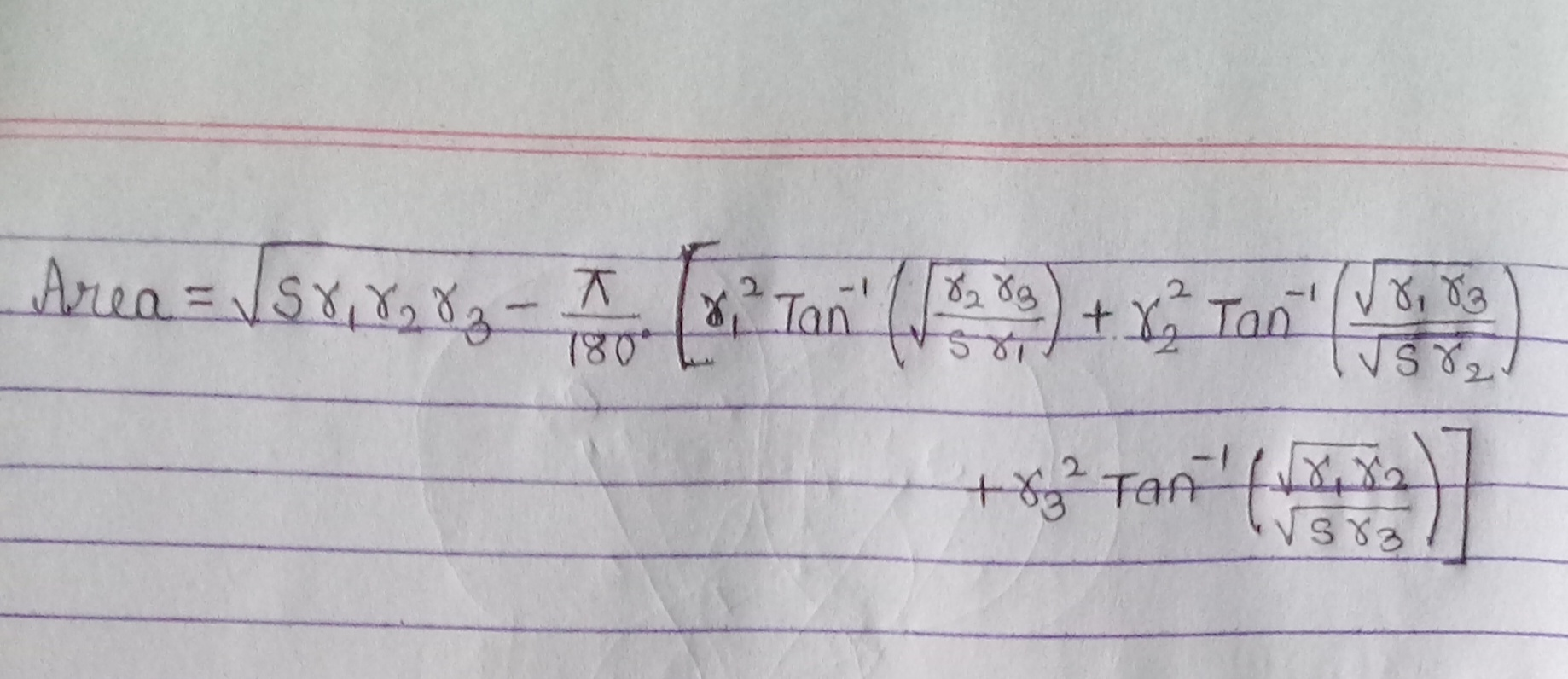I got it Where,s=r1+r2+r3

Incredibly! long formula

- 1 year ago

Ya,the earlier solution is longer than it.I'll try to short the formula

Can you describe the parts of this formula? Did you "use Heron's formula and then try to subtract the sectors"... If so I think there is a small mistake in Heron's formula, it's: $\sqrt{s (s-r_{1})(s-r_{2})(s-r_{3})}$. Anyways please describe how you got till this formula. Thanks!

- 1 year ago

@Mahdi Raza,check my solution

Wait…but the triangle’s sides aren’t $r_1,r_2,r_3$, and it is equilateral (tangent of circle from same point)

- 1 year ago

It might be the smallest among the radii of the circles, but we need more proof. :/

- 1 year ago

@Jeff Giff, @Mahdi Raza, @Kriti Kamal - the red area is not a triangle so don't confuse, it is a shape made of three arcs not three sides.

- 1 year ago

Yes, but I meant the smallest triangle containing the red area :)

- 1 year ago

I have considered them as arcs not as linesegmets :-)

Oic. My solution is different. I thought about the smallest triangle containing the red area :)

- 1 year ago

So my solution is $sizeof\triangle$containing red area $-sizeofARCH1,2,3$

- 1 year ago

Maybe I am wrong :D Sorry for being unhelpful :|

- 1 year ago

See my solution..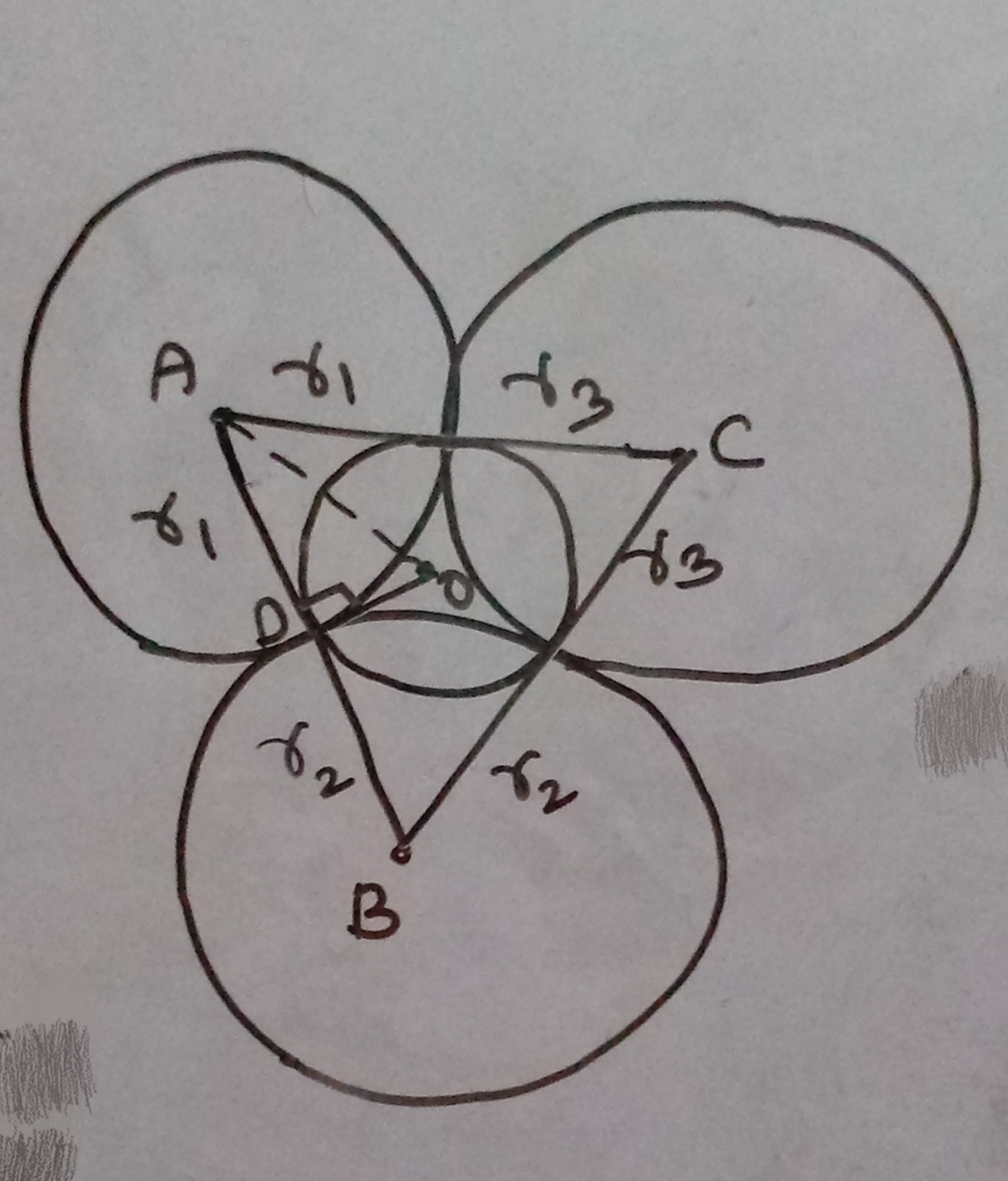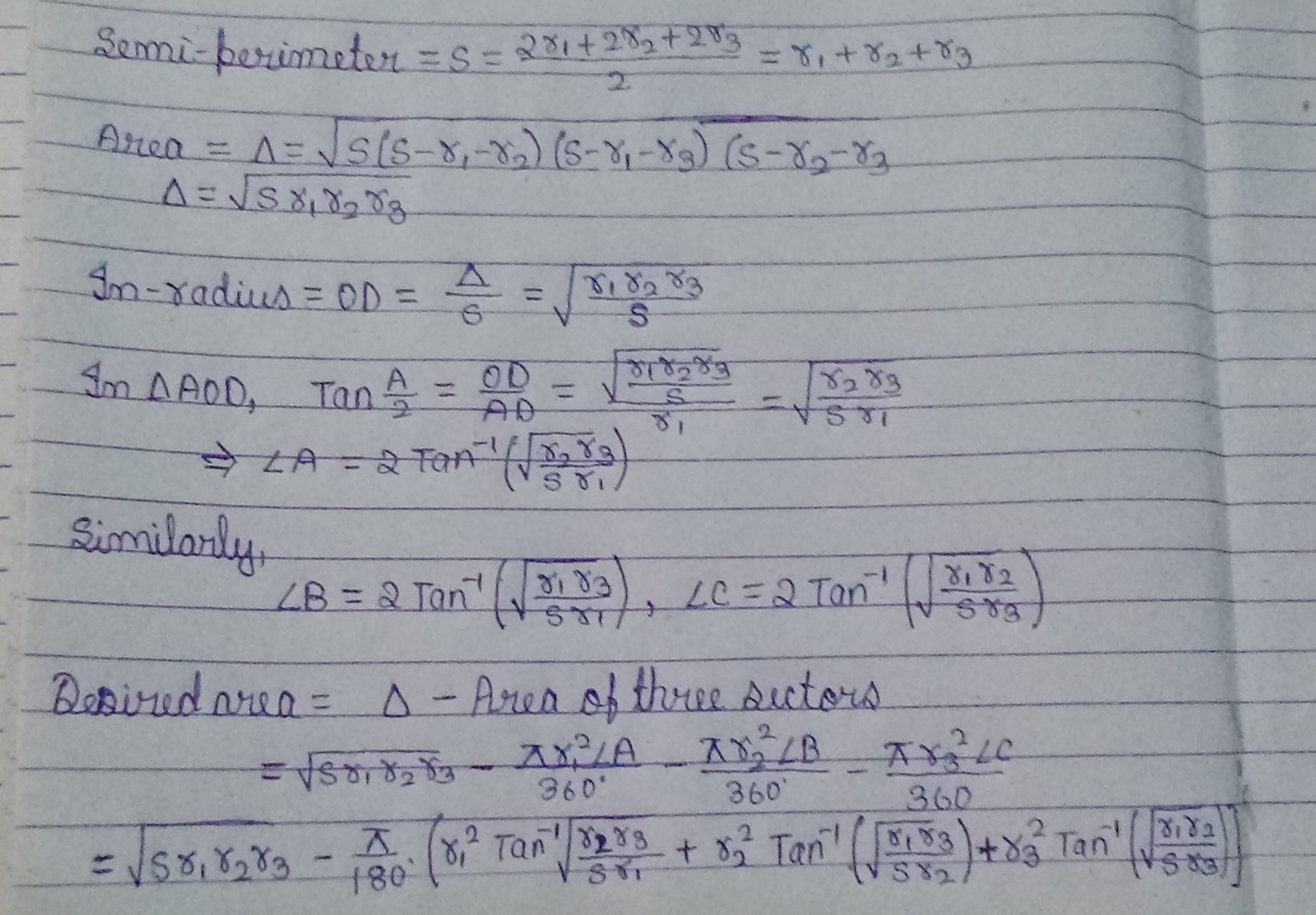Here,is my solution.Please comment if you find any mistake.Sorry for delay,it happens because tommorow my hands were burn by fire.

The angles can be calculated in sin inverse and cos inverse function

@Zakir Husain,can i send another version of solution

Sure!

- 1 year ago

The area of the triangle can be found using Zakir’s formula above:

$\frac12r_1^2\tan\angle A\sec\angle A$

Or any $x$ and $\theta$ pair with the relation above.

- 1 year ago

You are assuming that the perpendicular of $\overline{AB}$ at point $D$ will touch the point $C$, the same assumption you have to make to the perpendicular of $\overline{AC}$ and point $B$. Then only you can apply my formula to get the area of $\triangle ABC$. You first have to prove that these assumptions are true for all cases. Or you can build a new formula for this special case separately.

- 1 year ago

Aha! @Zakir Husain, your formula can be simplified as $\frac12x^2\sin \theta$, since $\tan \theta \sec \theta=\tan \theta \frac{1}{\cos \theta}=\frac{\frac{\sin \theta}{\cos \theta}}{\cos \theta}=\sin \theta$ :)

- 1 year ago

$\tan\theta\sec\theta=\dfrac{\sin\theta}{\cos\theta}\times\dfrac{1}{\cos\theta}=\red{\dfrac{\sin\theta}{\cos^2\theta}}$

- 1 year ago

Oops!

- 1 year ago

Slight improvement: express angles in radians. Then, the formula becomes $\sqrt{sr_1r_2r_3}-\sum _{cyc}r_1^2\tan^{-1}\sqrt{\frac{r_2r_3}{sr_1}}$

- 1 year ago